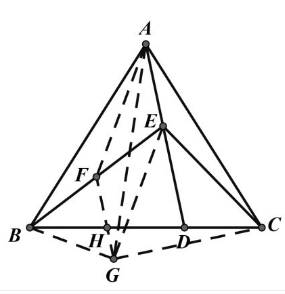【答案】

$\triangle A B F \cong \triangle C A E, \therefore \angle A F E=\angle C E D$,
$\angle B E D=2 \angle C E D, \therefore \angle A F E=\angle F A E, A E=F E$

$\therefore E G / / A F$, 四边形 $A F G E$ 是平行四边形。 $E G=A F=C E$,
$\therefore \triangle C A E \cong \triangle G A E$ 。
$\therefore A D$ 平分 $G C$, 又 $F G / / A D, D$ 是 $H C$ 中点。